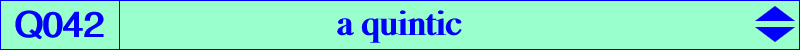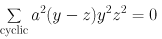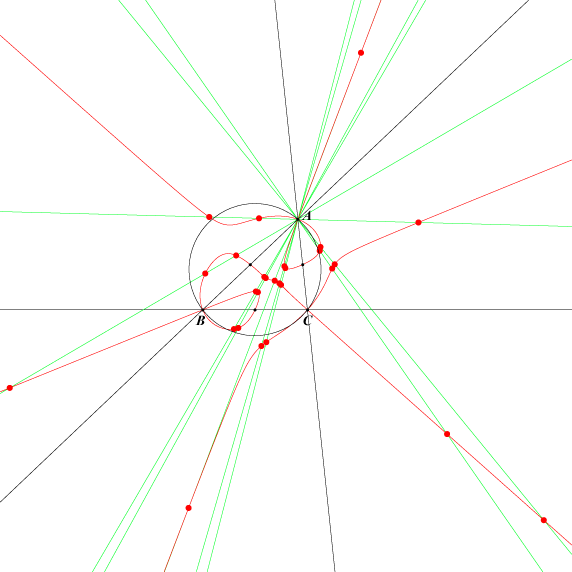X(1), X(2), X(13), X(14), X(88), X(3602), X(3603), X(3604) X(3602), X(3603), X(3604) are the isogonal conjugates of X(3273), X(3274), X(3275) Ia, Ib, Ic : excenters Ma, Mb, Mc : midpoints of ABC extraversions of X(88) common points of the circumcircle and the complement of the Kiepert hyperbola six equilateral anticevian points (see Table 14) (Edward Brisse) 27 points with barycentrics : a/sin((A+i 2pi)/3) : b/sin((B+j 2pi)/3) : c/sin((C+k 2pi)/3) with i, j, k in {-1, 0, 1}. See below.Q042 is the locus of point P such that : P, its isogonal conjugate P* and G/P are collinear (G/P is the center of the circum-conic with perspector P i.e. the G-Ceva conjugate of P). G, P and (P^2)* are collinear where (P^2)* is the isogonal conjugate of the barycentric square of P. A, B, C are double points with tangents the bisectors. The tangents at the midpoints Ma, Mb, Mc are the medians. The tangents at I, Ia, Ib, Ic and G concur at K. See a generalization of Q042 at the bottom of the page Q003. The isogonal transform of Q042 is the quartic Q043. See also Table 28 : cevian and anticevian points. Q042 contains the {X}-Anticevian points of any X on the Kiepert hyperbola. K754 is the locus of points whose polar conic in Q042 is a rectangular hyperbola. In other words, the cubic is the Laplacian of the quintic.Q042 and the "X(357) sinus points" These 27 points lie on three groups of nine lines passing through the points A, B, C. They are analogous to the perspectors of ABC and the 27 Morley triangles since the Cosinus are replaced by Sinus in their coordinates.